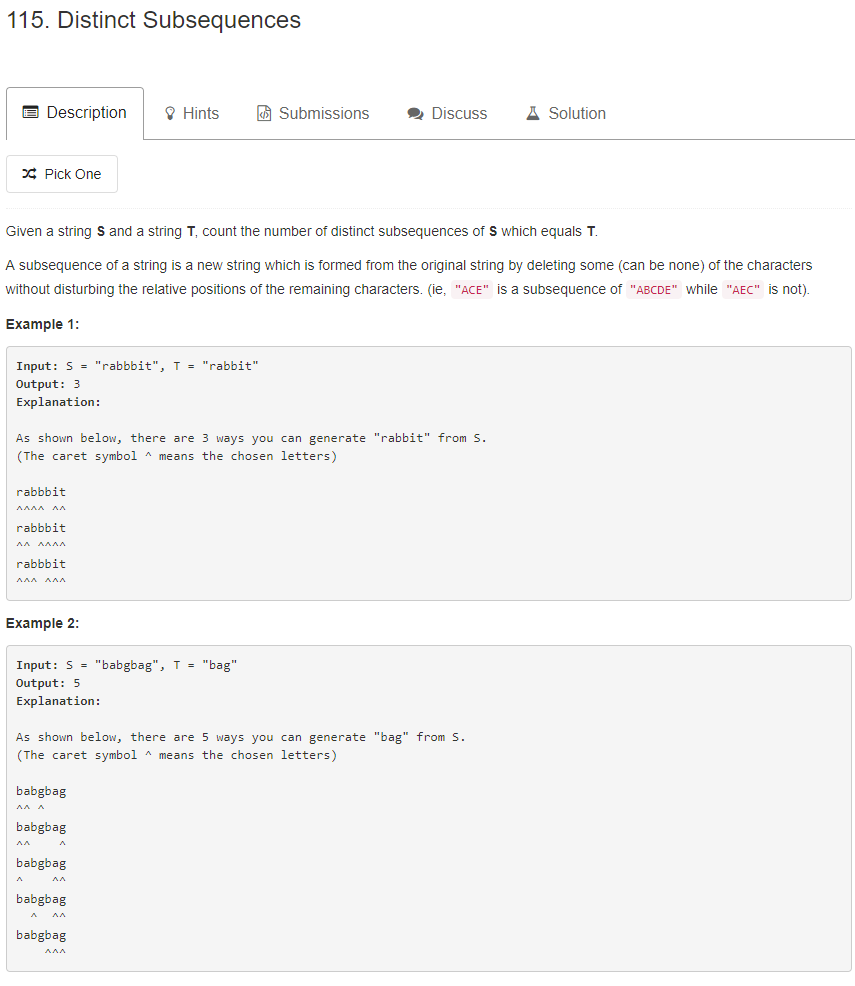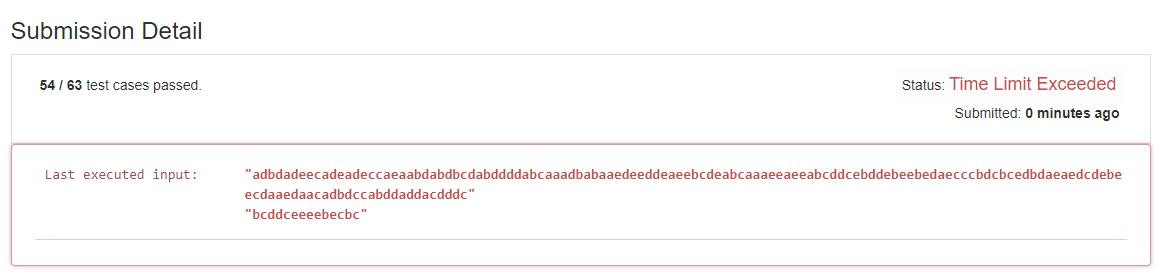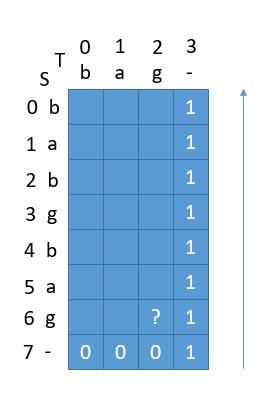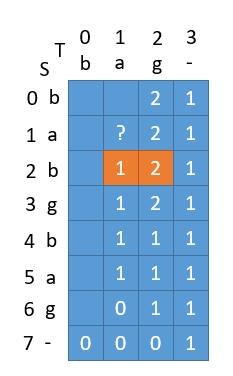# 题目描述（困难难度）# 解法一 递归之分治

S 中的每个字母就是两种可能选他或者不选他。我们用递归的常规思路，将大问题化成小问题，也就是分治的思想。

• S == T，需要知道两种情况

• S 中选择当前的字母，此时 S 跳过这个字母, T 也跳过一个字母。

去求 S[1，S_len - 1] 中能选出多少个 T[1，T_len - 1]，个数记为 n1

• S 不选当前的字母，此时S跳过这个字母，T 不跳过字母。

去求S[1，S_len - 1] 中能选出多少个 T[0，T_len - 1]，个数记为 n2

• S ！= T

S 只能不选当前的字母，此时S跳过这个字母， T 不跳过字母。

去求S[1，S_len - 1] 中能选出多少个 T[0，T_len - 1]，个数记为 n1

if(S == T){
n = n1 + n2;
}else{
n = n1;
}


public int numDistinct(String s, String t) {
return numDistinctHelper(s, 0, t, 0);
}

private int numDistinctHelper(String s, int s_start, String t, int t_start) {
int count = 0;
//当前字母相等
if (s.charAt(s_start) == t.charAt(t_start)) {
//从 S 选择当前的字母，此时 S 跳过这个字母, T 也跳过一个字母。
count = numDistinctHelper(s, s_start + 1, t, t_start + 1, map)
//S 不选当前的字母，此时 S 跳过这个字母，T 不跳过字母。
+ numDistinctHelper(s, s_start + 1, t, t_start,  map);
//当前字母不相等
}else{
//S 只能不选当前的字母，此时 S 跳过这个字母， T 不跳过字母。
count = numDistinctHelper(s, s_start + 1, t, t_start,  map);
}
return count;
}


public int numDistinct(String s, String t) {
return numDistinctHelper(s, 0, t, 0);
}

private int numDistinctHelper(String s, int s_start, String t, int t_start) {
//T 是空串，选法就是 1 种
if (t_start == t.length()) {
return 1;
}
//S 是空串，选法是 0 种
if (s_start == s.length()) {
return 0;
}
int count = 0;
//当前字母相等
if (s.charAt(s_start) == t.charAt(t_start)) {
//从 S 选择当前的字母，此时 S 跳过这个字母, T 也跳过一个字母。
count = numDistinctHelper(s, s_start + 1, t, t_start + 1)
//S 不选当前的字母，此时 S 跳过这个字母，T 不跳过字母。
+ numDistinctHelper(s, s_start + 1, t, t_start);
//当前字母不相等
}else{
//S 只能不选当前的字母，此时 S 跳过这个字母， T 不跳过字母。
count = numDistinctHelper(s, s_start + 1, t, t_start);
}
return count;
}mapkey的话就标识当前的递归，s_startt_start 联合表示，利用字符串 s_start + '@' + t_start

value的话就保存这次递归返回的count

public int numDistinct(String s, String t) {
HashMap<String, Integer> map = new HashMap<>();
return numDistinctHelper(s, 0, t, 0, map);
}

private int numDistinctHelper(String s, int s_start, String t, int t_start, HashMap<String, Integer> map) {
//T 是空串，选法就是 1 种
if (t_start == t.length()) {
return 1;
}
//S 是空串，选法是 0 种
if (s_start == s.length()) {
return 0;
}
String key = s_start + "@" + t_start;
//先判断之前有没有求过这个解
if (map.containsKey(key)) {
return map.get(key);
}
int count = 0;
//当前字母相等
if (s.charAt(s_start) == t.charAt(t_start)) {
//从 S 选择当前的字母，此时 S 跳过这个字母, T 也跳过一个字母。
count = numDistinctHelper(s, s_start + 1, t, t_start + 1, map)
//S 不选当前的字母，此时 S 跳过这个字母，T 不跳过字母。
+ numDistinctHelper(s, s_start + 1, t, t_start, map);
//当前字母不相等
}else{
//S 只能不选当前的字母，此时 S 跳过这个字母， T 不跳过字母。
count = numDistinctHelper(s, s_start + 1, t, t_start, map);
}
//将当前解放到 map 中
map.put(key, count);
return count;
}


# 解法二 递归之回溯

S串的当前字母和T串的当前字母相等，我们就可以选择S的当前字母，进入递归。

public int numDistinct3(String s, String t) {
numDistinctHelper(s, 0, t, 0);
}

private void numDistinctHelper(String s, int s_start, String t, int t_start) {
//当前字母相等，选中当前 S 的字母，s_start 后移一个
//选中当前 S 的字母，意味着和 T 的当前字母匹配，所以 t_start 后移一个
if (s.charAt(s_start) == t.charAt(t_start)) {
numDistinctHelper(s, s_start + 1, t, t_start + 1);
}
//出来以后，继续尝试不选择当前字母，s_start 后移一个，t_start 不后移
numDistinctHelper(s, s_start + 1, t, t_start);
}


• t_start == T_len，那么就意味着当前从S中选择的字母组成了T，此时就代表一种选法。我们可以用一个全局变量countcount计数此时就加一。然后return，返回到上一层继续寻求解。

• s_start == S_len，此时S到达了结尾，直接 return。

int count = 0;
public int numDistinct(String s, String t) {
numDistinctHelper(s, 0, t, 0);
return count;
}

private void numDistinctHelper(String s, int s_start, String t, int t_start) {
if (t_start == t.length()) {
count++;
return;
}
if (s_start == s.length()) {
return;
}
//当前字母相等，s_start 后移一个，t_start 后移一个
if (s.charAt(s_start) == t.charAt(t_start)) {
numDistinctHelper(s, s_start + 1, t, t_start + 1);
}
//出来以后，继续尝试不选择当前字母，s_start 后移一个，t_start 不后移
numDistinctHelper(s, s_start + 1, t, t_start);
}mapkey和之前一样，标识当前的递归，s_startt_start 联合表示，利用字符串 s_start + '@' + t_start

mapvalue的话？存什么呢。区别于解法一，我们每次都得到了当前条件下的count，然后存起来了。而现在我们只有一个全局变量，该怎么办呢？存全局变量count吗？

if (map.containsKey(key)) {
... ...
}


if (map.containsKey(key)) {
count += map.get(key);
return;
}


int count = 0;
public int numDistinct(String s, String t) {
HashMap<String, Integer> map = new HashMap<>();
numDistinctHelper(s, 0, t, 0, map);
return count;
}

private void numDistinctHelper(String s, int s_start, String t, int t_start,
HashMap<String, Integer> map) {
if (t_start == t.length()) {
count++;
return;
}
if (s_start == s.length()) {
return;
}
String key = s_start + "@" + t_start;
if (map.containsKey(key)) {
count += map.get(key);
return;
}
int count_pre = count;
//当前字母相等，s_start 后移一个，t_start 后移一个
if (s.charAt(s_start) == t.charAt(t_start)) {
numDistinctHelper(s, s_start + 1, t, t_start + 1, map);
}
//出来以后，继续尝试不选择当前字母，s_start 后移一个，t_start 不后移
numDistinctHelper(s, s_start + 1, t, t_start, map);

//将增量存起来
int count_increment = count - count_pre;
map.put(key, count_increment);
}


# 解法三 动态规划

//T 是空串，选法就是 1 种
if (t_start == t.length()) {
return 1;
}
//S 是空串，选法是 0 种
if (s_start == s.length()) {
return 0;
}


T 是空串或者 S 是空串，我们就直接可以返回结果了，接下来就是不停的出栈出栈，然后把结果通过递推关系取得。

m == S_len，意味着S是空串，此时dp[S_len][n]，n 取 0 到 T_len - 1的值都为 0

n == T_len，意味着T是空串，此时dp[m][T_len]，m 取 0 到 S_len的值都为 1

• S[s] == T[t]，当前字符相等，那就对应两种情况，选择S的当前字母和不选择S的当前字母

dp[s][t] = dp[s+1][t+1] + dp[s+1][t]

• S[s] != T[t]，只有一种情况，不选择S的当前字母

dp[s][t] = dp[s+1][t]

public int numDistinct(String s, String t) {
int s_len = s.length();
int t_len = t.length();
int[][] dp = new int[s_len + 1][t_len + 1];
//当 T 为空串时，所有的 s 对应于 1
for (int i = 0; i <= s_len; i++) {
dp[i][t_len] = 1;
}

//倒着进行，T 每次增加一个字母
for (int t_i = t_len - 1; t_i >= 0; t_i--) {
dp[s_len][t_i] = 0; // 这句可以省去，因为默认值是 0
//倒着进行，S 每次增加一个字母
for (int s_i = s_len - 1; s_i >= 0; s_i--) {
//如果当前字母相等
if (t.charAt(t_i) == s.charAt(s_i)) {
//对应于两种情况，选择当前字母和不选择当前字母
dp[s_i][t_i] = dp[s_i + 1][t_i + 1] + dp[s_i + 1][t_i];
//如果当前字母不相等
} else {
dp[s_i][t_i] = dp[s_i + 1][t_i];
}
}
}
return dp;
}


S = "babgbag", T = "bag"

T 为空串时，所有的 s 对应于 1。 S 为空串时，所有的 t 对应于 0。public int numDistinct(String s, String t) {
int s_len = s.length();
int t_len = t.length();
int[]dp = new int[s_len + 1];
for (int i = 0; i <= s_len; i++) {
dp[i] = 1;
}
//倒着进行，T 每次增加一个字母
for (int t_i = t_len - 1; t_i >= 0; t_i--) {
int pre = dp[s_len];
dp[s_len] = 0;
//倒着进行，S 每次增加一个字母
for (int s_i = s_len - 1; s_i >= 0; s_i--) {
int temp = dp[s_i];
if (t.charAt(t_i) == s.charAt(s_i)) {
dp[s_i] = dp[s_i + 1] + pre;
} else {
dp[s_i] = dp[s_i + 1];
}
pre = temp;
}
}
return dp;
}


public int numDistinct(String s, String t) {
int s_len = s.length();
int t_len = t.length();
int[] dp = new int[s_len + 1];
for (int i = 0; i <= s_len; i++) {
dp[i] = 1;
}
for (int t_i = 1; t_i <= t_len; t_i++) {
int pre = dp;
dp = 0;
for (int s_i = 1; s_i <= s_len; s_i++) {
int temp = dp[s_i];
if (t.charAt(t_i - 1) == s.charAt(s_i - 1)) {
dp[s_i] = dp[s_i - 1] + pre;
} else {
dp[s_i] = dp[s_i - 1];
}
pre = temp;
}
}
return dp[s_len];
}